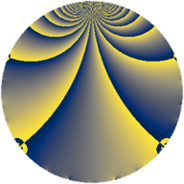Properties

 Label 1050.3.vLevel $1050$ Weight $3$ Character orbit 1050.v Rep. character $\chi_{1050}(139,\cdot)$ Character field $\Q(\zeta_{10})$ Dimension $320$ Sturm bound $720$

Related objects

Defining parameters

 Level: $$N$$ $$=$$ $$1050 = 2 \cdot 3 \cdot 5^{2} \cdot 7$$ Weight: $$k$$ $$=$$ $$3$$ Character orbit: $$[\chi]$$ $$=$$ 1050.v (of order $$10$$ and degree $$4$$) Character conductor: $$\operatorname{cond}(\chi)$$ $$=$$ $$175$$ Character field: $$\Q(\zeta_{10})$$ Sturm bound: $$720$$

Dimensions

The following table gives the dimensions of various subspaces of $$M_{3}(1050, [\chi])$$.

Total New Old
Modular forms 1952 320 1632
Cusp forms 1888 320 1568
Eisenstein series 64 0 64

Trace form

 $$320 q + 160 q^{4} - 240 q^{9} + O(q^{10})$$ $$320 q + 160 q^{4} - 240 q^{9} + 120 q^{11} + 24 q^{15} - 320 q^{16} - 40 q^{22} - 200 q^{23} - 24 q^{25} - 40 q^{28} + 120 q^{29} + 96 q^{30} - 212 q^{35} + 480 q^{36} - 120 q^{42} + 160 q^{44} - 120 q^{46} - 80 q^{49} + 96 q^{50} + 120 q^{53} + 192 q^{60} + 180 q^{63} + 640 q^{64} - 368 q^{65} - 24 q^{70} + 160 q^{71} - 320 q^{74} + 320 q^{79} - 720 q^{81} - 480 q^{86} - 320 q^{88} - 380 q^{91} - 280 q^{95} + 320 q^{98} - 240 q^{99} + O(q^{100})$$

Decomposition of $$S_{3}^{\mathrm{new}}(1050, [\chi])$$ into newform subspaces

The newforms in this space have not yet been added to the LMFDB.

Decomposition of $$S_{3}^{\mathrm{old}}(1050, [\chi])$$ into lower level spaces

$$S_{3}^{\mathrm{old}}(1050, [\chi]) \cong$$ $$S_{3}^{\mathrm{new}}(175, [\chi])$$$$^{\oplus 4}$$$$\oplus$$$$S_{3}^{\mathrm{new}}(350, [\chi])$$$$^{\oplus 2}$$$$\oplus$$$$S_{3}^{\mathrm{new}}(525, [\chi])$$$$^{\oplus 2}$$